Hello, Guest!
 Instructional Focus DocumentGeometry
 TITLE : Unit 11: Engineering Design SUGGESTED DURATION : 10 days

#### Unit Overview

Introduction
This is a project-based unit where students apply bundled student expectations that address constructing and analyzing models and making conjectures about geometric relationships in engineering design of architectural structures. Concepts are incorporated into both mathematical and real-world problem situations. According to the Texas Education Agency, mathematical process standards including application, tools and techniques, communication, representations, relationships, and justifications should be integrated (when applicable) with content knowledge and skills so that students are prepared to use mathematics in everyday life, society, and the workplace.

Prior to this Unit
In Units 01, 03, and 04, students investigated axiomatic systems and applied logical arguments to justify conjectures. In Units 05 – 09, students used models and diagrams to illustrate geometric relationships and solve problems. In Unit 02, images are created using rigid and non-rigid transformations. In Unit 09, students investigated and applied proportional and non-proportional dimensional change. In Unit 02, students determined the slope and equation for parallel and perpendicular lines. In Units 04 and 05, students investigated relationships in congruent triangles, similar triangles, and right triangles. In Units 08 and 09, students investigated and determined measurements in two- and three-dimensional figures, including area and volume. In Unit 10, students determined probability based on area in contextual problems.

During this Unit
Students apply prior knowledge to construct a three-dimensional structure and analyze the structure using two-dimensional views. Student groups research and select a structure composed of polygons and circles that meets at least five specified geometric elements. Students construct a three-dimensional scale model of the structure with lengths labeled to the nearest centimeter. Students draw two-dimensional diagrams of the top, front, and side views of the scale model, labeling all lengths to the nearest centimeter and all angles to the nearest degree. Students determine equations of the lines that represent each edge of the diagrams of the top, front, and side views of the scale model that could be used to create blueprints in a computer graphics software program. Students calculate the exposed surface area and volume of the scale model and summarize how the surface area and volume of the scale model could be used to find the surface area and volume of the original structure in square feet and cubic feet, respectively. Students create a list of at least three conjectures about the geometric relationships in the structure and verify these conjectures using measurement, deductive reasoning, coordinate geometry, or other techniques.

Other considerations: Reference the Mathematics COVID-19 Gap Implementation Tool HS Geometry

After this Unit
Students will continue to use these concepts in subsequent courses in mathematics. Students will also apply these concepts in possible career choices and everyday situations.

This unit is supporting the development of Texas College and Career Readiness Standards (TxCCRS): I. Numeric Reasoning B1, C1; II. Algebraic Reasoning A1, D1, D2; III. Geometric and Spatial Reasoning A2, A3, B1, B2, C1, D1, D2, D3; V. Statistical Reasoning A1, C2; VII. Problem Solving and Reasoning A1, A2, A3, A4, A5, B1, C1, C2, D1, D2; VIII. Communication and Representation A1, A2, A3, B1, B2, C1, C2, C3; IX. Connections A1, A2, B1, B2, B3.

Research
According to The National Council of Teachers of Mathematics (2000) this approach to studying quadrilaterals supports the need for all students to be able to analyze characteristics and properties of geometric shapes and develop mathematical arguments about geometric relationships. Students in grades 9 – 12 should specifically be able to investigate relationships among classes of two-dimensional geometric objects, make and test conjectures about them, and solve problems. At the conclusion of this unit, students are asked to create a graphic organizer to help classify quadrilaterals. The TxCCRS cites many skills related to the communication and representation of mathematical ideas. According to the National Council of Teachers of Mathematics (2009) in Focus in High School Mathematics: Reasoning and Sense Making, the key elements of reasoning and sense making with geometry must include multiple representations of functions. In this unit, students gather data from geometric figures and organize this information into tables, graphs or diagrams. This leads to the development of symbolic expressions and verbal descriptions. A variety of representations helps make relationships more understandable to more students than working with symbolic representations alone. These approaches serve as the basis for this unit on polygons and circle. TxCCRS cites many skills related to the communication and representation of mathematical ideas. According to the National Council of Teachers of Mathematics, using diagrams and constructions to interpret and communicate geometric relationships is essential in geometry. Using definitions of figures to characterize figures in terms of their properties is another essential in geometry. In geometry, the “proving process involves working with diagrams, variation and invariance, conjectures, and definitions.” (2012, p. 92)

National Council of Teachers of Mathematics. (2000). Principles and standards for school mathematics. Reston, VA: National Council of Teachers of Mathematics, Inc.
National Council of Teachers of Mathematics. (2009). Focus in High School Mathematics: Reasoning and Sense Making. Reston, VA: National Council of Teachers of Mathematics, Inc.
National Council of Teachers of Mathematics. (2012). Developing essential understanding of Geometry for Teaching Mathematics in Grades 9 – 12. Reston, VA: National Council of Teachers of Mathematics, Inc.
Texas Education Agency & Texas Higher Education Coordinating Board. (2009). Texas college and career readiness standards. Retrieved from http://www.thecb.state.tx.us/index.cfm?objectid=E21AB9B0-2633-11E8-BC500050560100A9

 Quantitative relationships model problem situations efficiently and can be used to make generalizations, predictions, and critical judgements in everyday life. What patterns exist within different types of quantitative relationships and where are they found in everyday life? Why is the ability to model quantitative relationships in a variety of ways essential to solving problems in everyday life? Geometric, spatial, and measurement reasoning are foundational to visualizing, analyzing, and applying relationships within and between scale, shapes, quantities, and spatial relations in everyday life. Why is developing geometric, spatial, and measurement reasoning essential? How does geometric, spatial, and measurement reasoning affect how one sees and works in the world? Logical reasoning can be used to make sense of claims, determine their validity, and construct and communicate arguments. Why is developing logical reasoning in mathematics important and how does this reasoning influence decision making in everyday life? What elements of logical reasoning influence the truth of a statement? How is logical reasoning used to uncover truths and/or make sense of, construct, and determine the validity of arguments and claims?
Unit Understandings
and Questions
Overarching Concepts
and Unit Concepts
Performance Assessment(s)
• Understanding and working with variance and invariance within geometry builds flexible algebraic and geometric reasoning and deepens understanding of intrinsic properties of geometric relationships.
• What invariant (unchanging) and variant (changing) relationships exist within and between geometric figures?
• How does scaling measurements of geometric figures demonstrate variance and invariance?
• Deductive reasoning can be used to determine the validity of a conditional statement and its related statements and conjectures about geometric relationships in order to support or refute mathematical claims through the process of proving.
• How is deductive reasoning used to understand, prove, and apply geometric conjectures?
• What structures and processes can be used to prove geometric relationships?
• Accurate representations, models, or diagrams within a geometric system allows for visualizing, illustrating, and analyzing geometric relationships to aid in making and validating conjectures about those geometric relationships and is central to geometric thinking.
• What types of problem situations represent relationships in geometric figures, including composite figures?
• How can representations and appropriate geometric language be used to effectively communicate and illustrate geometric relationships?
• What tools and processes can be used to investigate geometric figures, including the building of scaled models?
• What conjectures can be made and validated by exploring the patterns and properties of geometric figures?
• Attributes and quantifiable measures of geometric figures can be generalized to describe, determine, and represent algebraic and geometric relationships and be applied to solve problem situations.
• How can understanding relationships in geometric figures, including composite figures, be applied when solving problem situations?
• How can measurable attributes be distinguished and described in order to generalize geometric relationships of geometric figures?
• What processes can be used to determine the linear, area, and volume measures of geometric figures, including composite figures?
• Coordinate and Transformational Geometry; Similarity, Proof, and Trigonometry; Two-Dimensional and Three-Dimensional Figures; Circles
• Circles
• Geometric Relationships
• Geometric Representations
• Two-Dimensional Coordinate Systems
• Patterns, Operations, and Properties
• Transformations
• Triangle Relationships
• Logical Arguments and Constructions, Proof and Congruence
• Deductive Reasoning
• Geometric Relationships
• Geometric Representations
• Associated Mathematical Processes
• Application
• Problem Solving Model
• Tools and Techniques
• Communication
• Representations
• Relationships
• Justification
 Assessment information provided within the TEKS Resource System are examples that may, or may not, be used by your child’s teacher. In accordance with section 26.006 (2) of the Texas Education Code, "A parent is entitled to review each test administered to the parent’s child after the test is administered." For more information regarding assessments administered to your child, please visit with your child’s teacher.

#### MISCONCEPTIONS / UNDERDEVELOPED CONCEPTS

• None identified

#### Unit Vocabulary

Related Vocabulary:

 Arc length of a circle Base angles of a triangle Cone Congruent angles Congruent figures Congruent segments Corresponding angles Cylinder Diagonals of quadrilaterals Distance Distance formula of a line segment Midpoint Midpoint formula of a line segment Non-rigid transformation Parallel lines Parallelogram Perpendicular lines Polygon Prism Proofs Proportional sides Pyramid Pythagorean Theorem Pythagorean triples Quadrilateral Rectangle Regular polygon Rhombus Rigid transformation Right triangles Sector of a circle Semicircle Similar figures Slope Slope formula of a line segment Special right triangles Sphere Square Three-dimensional figures Three-dimensional proportional change Three-dimensional non-proportional change Transformations Trapezoid Trigonometric ratios Two-dimensional figures
Unit Assessment Items System Resources Other Resources

Show this message:

Unit Assessment Items that have been published by your district may be accessed through Search All Components in the District Resources tab. Assessment items may also be found using the Assessment Center if your district has granted access to that tool.

System Resources may be accessed through Search All Components in the District Resources Tab.

Texas Higher Education Coordinating Board – Texas College and Career Readiness Standards

Texas Education Agency – Mathematics Curriculum

Texas Education Agency – STAAR Mathematics Resources

Texas Education Agency Texas Gateway – Revised Mathematics TEKS: Vertical Alignment Charts

Texas Education Agency Texas Gateway – Mathematics TEKS: Supporting Information

Texas Education Agency Texas Gateway – Interactive Mathematics Glossary

Texas Education Agency Texas Gateway – Resources Aligned to Geometry Mathematics TEKS

Texas Instruments – Graphing Calculator Tutorials

TAUGHT DIRECTLY TEKS

TEKS intended to be explicitly taught in this unit.

TEKS/SE Legend:

• Knowledge and Skills Statements (TEKS) identified by TEA are in italicized, bolded, black text.
• Student Expectations (TEKS) identified by TEA are in bolded, black text.
• Portions of the Student Expectations (TEKS) that are not included in this unit but are taught in previous or future units are indicated by a strike-through.

Specificity Legend:

• Supporting information / clarifications (specificity) written by TEKS Resource System are in blue text.
• Unit-specific clarifications are in italicized, blue text.
• Information from Texas Education Agency (TEA), Texas College and Career Readiness Standards (TxCCRS), Texas Response to Curriculum Focal Points (TxRCFP) is labeled.
• A Partial Specificity label indicates that a portion of the specificity not aligned to this unit has been removed.
TEKS# SE# TEKS SPECIFICITY
G.1 Mathematical process standards. The student uses mathematical processes to acquire and demonstrate mathematical understanding. The student is expected to:
G.1A Apply mathematics to problems arising in everyday life, society, and the workplace.

Apply

MATHEMATICS TO PROBLEMS ARISING IN EVERYDAY LIFE, SOCIETY, AND THE WORKPLACE

Including, but not limited to:

• Mathematical problem situations within and between disciplines
• Everyday life
• Society
• Workplace

Note(s):

• The mathematical process standards may be applied to all content standards as appropriate.
• TxCCRS:
• VII.D. Problem Solving and Reasoning – Real-world problem solving
• VII.D.1. Interpret results of the mathematical problem in terms of the original real-world situation.
• IX.A. Connections – Connections among the strands of mathematics
• IX.A.1. Connect and use multiple key concepts of mathematics in situations and problems.
• IX.A.2. Connect mathematics to the study of other disciplines.
• IX.B. Connections – Connections of mathematics to nature, real-world situations, and everyday life
• IX.B.1. Use multiple representations to demonstrate links between mathematical and real-world situations.
• IX.B.2. Understand and use appropriate mathematical models in the natural, physical, and social sciences.
• IX.B.3. Know and understand the use of mathematics in a variety of careers and professions.
G.1B Use a problem-solving model that incorporates analyzing given information, formulating a plan or strategy, determining a solution, justifying the solution, and evaluating the problem-solving process and the reasonableness of the solution.

Use

A PROBLEM-SOLVING MODEL THAT INCORPORATES ANALYZING GIVEN INFORMATION, FORMULATING A PLAN OR STRATEGY, DETERMINING A SOLUTION, JUSTIFYING THE SOLUTION, AND EVALUATING THE PROBLEM-SOLVING PROCESS AND THE REASONABLENESS OF THE SOLUTION

Including, but not limited to:

• Problem-solving model
• Analyze given information
• Formulate a plan or strategy
• Determine a solution
• Justify the solution
• Evaluate the problem-solving process and the reasonableness of the solution

Note(s):

• The mathematical process standards may be applied to all content standards as appropriate.
• TxCCRS:
• I.B. Numeric Reasoning – Number sense and number concepts
• I.B.1. Use estimation to check for errors and reasonableness of solutions.
• V.A. Statistical Reasoning – Design a study
• V.A.1. Formulate a statistical question, plan an investigation, and collect data.
• VII.A. Problem Solving and Reasoning – Mathematical problem solving
• VII.A.1. Analyze given information.
• VII.A.2. Formulate a plan or strategy.
• VII.A.3. Determine a solution.
• VII.A.4. Justify the solution.
• VII.A.5. Evaluate the problem-solving process.
• VII.D. Problem Solving and Reasoning – Real-world problem solving
• VII.D.2. Evaluate the problem-solving process.
G.1C Select tools, including real objects, manipulatives, paper and pencil, and technology as appropriate, and techniques, including mental math, estimation, and number sense as appropriate, to solve problems.

Select

TOOLS, INCLUDING REAL OBJECTS, MANIPULATIVES, PAPER AND PENCIL, AND TECHNOLOGY AS APPROPRIATE, AND TECHNIQUES, INCLUDING MENTAL MATH, ESTIMATION, AND NUMBER SENSE AS APPROPRIATE, TO SOLVE PROBLEMS

Including, but not limited to:

• Appropriate selection of tool(s) and techniques to apply in order to solve problems
• Tools
• Real objects
• Manipulatives
• Paper and pencil
• Technology
• Techniques
• Mental math
• Estimation
• Number sense

Note(s):

• The mathematical process standards may be applied to all content standards as appropriate.
• TxCCRS:
• I.B. Numeric Reasoning – Number sense and number concepts
• I.B.1. Use estimation to check for errors and reasonableness of solutions.
• V.C. Statistical Reasoning – Analyze, interpret, and draw conclusions from data
• V.C.2. Analyze relationships between paired data using spreadsheets, graphing calculators, or statistical software.
G.1D Communicate mathematical ideas, reasoning, and their implications using multiple representations, including symbols, diagrams, graphs, and language as appropriate.

Communicate

MATHEMATICAL IDEAS, REASONING, AND THEIR IMPLICATIONS USING MULTIPLE REPRESENTATIONS, INCLUDING SYMBOLS, DIAGRAMS, GRAPHS, AND LANGUAGE AS APPROPRIATE

Including, but not limited to:

• Mathematical ideas, reasoning, and their implications
• Multiple representations, as appropriate
• Symbols
• Diagrams
• Graphs
• Language

Note(s):

• The mathematical process standards may be applied to all content standards as appropriate.
• TxCCRS:
• II.D. Algebraic Reasoning – Representing relationships
• II.D.1. Interpret multiple representations of equations, inequalities, and relationships.
• II.D.2. Convert among multiple representations of equations, inequalities, and relationships.
• VIII.A. Communication and Representation – Language, terms, and symbols of mathematics
• VIII.A.1. Use mathematical symbols, terminology, and notation to represent given and unknown information in a problem.
• VIII.A.2. Use mathematical language to represent and communicate the mathematical concepts in a problem.
• VIII.A.3. Use mathematical language for reasoning, problem solving, making connections, and generalizing.
• VIII.B. Communication and Representation – Interpretation of mathematical work
• VIII.B.1. Model and interpret mathematical ideas and concepts using multiple representations.
• VIII.B.2. Summarize and interpret mathematical information provided orally, visually, or in written form within the given context.
• VIII.C. Communication and Representation – Presentation and representation of mathematical work
• VIII.C.1. Communicate mathematical ideas, reasoning, and their implications using symbols, diagrams, models, graphs, and words.
• VIII.C.2. Create and use representations to organize, record, and communicate mathematical ideas.
• VIII.C.3. Explain, display, or justify mathematical ideas and arguments using precise mathematical language in written or oral communications.
• IX.B. Connections – Connections of mathematics to nature, real-world situations, and everyday life
• IX.B.1. Use multiple representations to demonstrate links between mathematical and real-world situations.
G.1E Create and use representations to organize, record, and communicate mathematical ideas.

Create, Use

REPRESENTATIONS TO ORGANIZE, RECORD, AND COMMUNICATE MATHEMATICAL IDEAS

Including, but not limited to:

• Representations of mathematical ideas
• Organize
• Record
• Communicate
• Evaluation of the effectiveness of representations to ensure clarity of mathematical ideas being communicated
• Appropriate mathematical vocabulary and phrasing when communicating mathematical ideas

Note(s):

• The mathematical process standards may be applied to all content standards as appropriate.
• TxCCRS:
• VIII.B. Communication and Representation – Interpretation of mathematical work
• VIII.B.1. Model and interpret mathematical ideas and concepts using multiple representations.
• VIII.B.2. Summarize and interpret mathematical information provided orally, visually, or in written form within the given context.
• VIII.C. Communication and Representation – Presentation and representation of mathematical work
• VIII.C.1. Communicate mathematical ideas, reasoning, and their implications using symbols, diagrams, models, graphs, and words.
• VIII.C.2. Create and use representations to organize, record, and communicate mathematical ideas.
G.1F Analyze mathematical relationships to connect and communicate mathematical ideas.

Analyze

MATHEMATICAL RELATIONSHIPS TO CONNECT AND COMMUNICATE MATHEMATICAL IDEAS

Including, but not limited to:

• Mathematical relationships
• Connect and communicate mathematical ideas
• Conjectures and generalizations from sets of examples and non-examples, patterns, etc.
• Current knowledge to new learning

Note(s):

• The mathematical process standards may be applied to all content standards as appropriate.
• TxCCRS:
• VII.A. Problem Solving and Reasoning – Mathematical problem solving
• VII.A.1. Analyze given information.
• VIII.A. Communication and Representation – Language, terms, and symbols of mathematics
• VIII.A.1. Use mathematical symbols, terminology, and notation to represent given and unknown information in a problem.
• VIII.A.2. Use mathematical language to represent and communicate the mathematical concepts in a problem.
• VIII.A.3. Use mathematical language for reasoning, problem solving, making connections, and generalizing.
•  VIII.B. Communication and Representation – Interpretation of mathematical work
• VIII.B.1. Model and interpret mathematical ideas and concepts using multiple representations.
• VIII.C. Communication and Representation – Presentation and representation of mathematical work
• VIII.C.1. Communicate mathematical ideas, reasoning, and their implications using symbols, diagrams, models, graphs, and words.
• VIII.C.2. Create and use representations to organize, record, and communicate mathematical ideas.
• VIII.C.3. Explain, display, or justify mathematical ideas and arguments using precise mathematical language in written or oral communications.
• IX.A. Connections – Connections among the strands of mathematics
• IX.A.1. Connect and use multiple key concepts of mathematics in situations and problems.
• IX.A.2. Connect mathematics to the study of other disciplines.
G.1G Display, explain, and justify mathematical ideas and arguments using precise mathematical language in written or oral communication.

Display, Explain, Justify

MATHEMATICAL IDEAS AND ARGUMENTS USING PRECISE MATHEMATICAL LANGUAGE IN WRITTEN OR ORAL COMMUNICATION

Including, but not limited to:

• Mathematical ideas and arguments
• Validation of conclusions
• Displays to make work visible to others
• Diagrams, visual aids, written work, etc.
• Explanations and justifications
• Precise mathematical language in written or oral communication

Note(s):

• The mathematical process standards may be applied to all content standards as appropriate.
• TxCCRS:
• VII.A. Problem Solving and Reasoning – Mathematical problem solving
• VII.A.4. Justify the solution.
• VII.B. Problem Solving and Reasoning – Proportional reasoning
• VII.B.1. Use proportional reasoning to solve problems that require fractions, ratios, percentages, decimals, and proportions in a variety of contexts using multiple representations.
• VII.C. Problem Solving and Reasoning – Logical reasoning
• VII.C.1. Develop and evaluate convincing arguments.
• VIII.A. Communication and Representation – Language, terms, and symbols of mathematics
• VIII.A.3. Use mathematical language for reasoning, problem solving, making connections, and generalizing.
• VIII.B. Communication and Representation – Interpretation of mathematical work
• VIII.B.1. Model and interpret mathematical ideas and concepts using multiple representations.
• VIII.B.2. Summarize and interpret mathematical information provided orally, visually, or in written form within the given context.
• VIII.C. Communication and Representation – Presentation and representation of mathematical work
• VIII. C.3. Explain, display, or justify mathematical ideas and arguments using precise mathematical language in written or oral communications.
G.2 Coordinate and transformational geometry. The student uses the process skills to understand the connections between algebra and geometry and uses the one- and two-dimensional coordinate systems to verify geometric conjectures. The student is expected to:
G.2C Determine an equation of a line parallel or perpendicular to a given line that passes through a given point.

Determine

AN EQUATION OF A LINE PARALLEL TO A GIVEN LINE THAT PASSES THROUGH A GIVEN POINT

Including, but not limited to:

• Parallel lines – lines in the same plane that never intersect. Slopes of parallel lines are equal, my2 = my1.
• Equation of a line
• Slope-intercept form, y = mx + b where m represents slope and b represents the y-intercept
• Point-slope form, yy1 = m(xx1) where m represents slope and (x1, y1) represents the given point
• Standard form, Ax + By = C where the slope, m = –• Determination of the equation of a line given a slope and y-intercept
• Determination of the equation of a line given a graph
• Determination of the equation of a line given a slope and a point
• Determination of the equation of a line given two points

Determine

AN EQUATION OF A LINE PERPENDICULAR TO A GIVEN LINE THAT PASSES THROUGH A GIVEN POINT

Including, but not limited to:

• Perpendicular lines – lines that intersect at a 90° angle to form right angles. Slopes of perpendicular lines are opposite reciprocals, my2 = –.
• Equation of a line
• Slope-intercept form, y = mx + b where m represents slope and b represents the y-intercept
• Point-slope form, yy1 = m(xx1) where m represents slope and (x1, y1) represents the given point
• Standard form, Ax + By = C where the slope, m = –• Determination of the equation of a line given a slope and y-intercept
• Determination of the equation of a line given a graph
• Determination of the equation of a line given a slope and a point
• Determination of the equation of a line given two points

Note(s):

• Grades 7 and 8 represented linear non-proportional situations using multiple representations, including y = mx + b, where b ≠ 0.
• Algebra I addressed determining equations of lines using point-slope form, slope intercept form, and standard form.
• Algebra I wrote equations of lines that contain a given point and are parallel or perpendicular to a given line.
• Various mathematical process standards will be applied to this student expectation as appropriate.
• TxCCRS
• III.C. Geometric and Spatial Reasoning – Connections between geometry and other mathematical content strands
• III.C.1. Make connections between geometry and algebraic equations.
• IX.A. Connections – Connections among the strands of mathematics
• IX.A.1. Connect and use multiple key concepts of mathematics in situations and problems.
G.3 Coordinate and transformational geometry. The student uses the process skills to generate and describe rigid transformations (translation, reflection, and rotation) and non-rigid transformations (dilations that preserve similarity and reductions and enlargements that do not preserve similarity). The student is expected to:
G.3A Describe and perform transformations of figures in a plane using coordinate notation.

Describe, Perform

TRANSFORMATIONS OF FIGURES IN A PLANE USING COORDINATE NOTATION

Including, but not limited to:

• Transformation – one to one mapping of points in a plane such that each point in the pre-image has a unique image and each point in the image has a pre-image
• Rigid transformation (isometric) – a transformation that preserves the size and shape of a figure
• Translation – rigid transformation moving all points in a geometric figure the same distance and the same direction
• Representations in coordinate notation: (x, y) → (x + a, y + b), a = horizontal shift, b = vertical shift
• Reflection – rigid transformation in which each point in a geometric figure is at an equal distance on the opposite side of a given line (line of symmetry)
• Reflection across the x-axis (x, y) → (x, –y)
• Reflection across the y-axis (x, y) → (–x, y)
• Reflection across the line y = x (x, y) → (y, x)
• Reflection across the line y = –x (x, y) → (–y, –x)
• Rotation – a rigid transformation where each point on the figure is rotated about a given point
• Rotations around the origin
• Rotation of 90º counterclockwise around the origin: (x, y) → (–y, x)
• Same as a rotation of 270º clockwise around the origin: (x, y) → (–y,x)
• Rotation of 180º counterclockwise around the origin: (x, y) → (–x, –y)
• Same as a rotation of 180º clockwise around the origin: (x, y) → (–x, –y)
• Rotation of 270º counterclockwise around the origin: (x, y) → (y, –x)
• Same as a rotation of 90º clockwise around the origin: (x, y)  → (y, –x)
• Rotation of 360º around the origin: (x, y) → (x, y)
• Rotation around a given point other than the origin
• Apply a translation that moves the point of rotation to the origin and translate the figure using the same translation
• Rotate the figure about the origin
• Translate the point of rotation to its original position by the opposite translation and apply the same translation to the rotated figure
• Non-Rigid transformation (non-isometric) – a transformation that preserves similarity and reductions and enlargements that do not preserve similarity
• Dilation – a similarity transformation in which a figure is enlarged or reduced using a scale factor and a center of dilation
• Representations in coordinate notation: (x, y) → (kx, ky), k = scale factor when the center of dilation is the origin
• Representations in coordinate notation: (x, y) → (k(xa) + a, k(yb) + b), k = scale factor, (a, b) is the center of dilation

Note(s):

• Grade 8 introduced transformations including translations, reflections, rotations, and dilations.
• Grade 8 introduced dilations with the origin as the center.
• Grade 8 generalized the properties of orientation and congruence of rotations, reflections, translations, and dilations of two-dimensional shapes on a coordinate plane.
• Grade 8 differentiated between transformations that preserved congruence and those that did not.
• Grade 8 introduced using an algebraic representation to explain the effect of a given positive rational scale factor applied to two-dimensional figures on a coordinate plane with the origin as the center of dilation.
• Various mathematical process standards will be applied to this student expectation as appropriate.
• TxCCRS
• III.B. Geometric and Spatial Reasoning – Transformations and symmetry
• III.B.1. Identify transformations and symmetries of figures.
• III.B.2. Use transformations to investigate congruence, similarity, and symmetries of figures.
• III.C. Geometric and Spatial Reasoning – Connections between geometry and other mathematical content strands
• III.C.1. Make connections between geometry and algebraic equations.
• VIII.C. Communication and Representation – Presentation and representation of mathematical work
• VIII.C.3. Explain, display, or justify mathematical ideas and arguments using precise mathematical language in written or oral communications.
G.5 Logical argument and constructions. The student uses constructions to validate conjectures about geometric figures. The student is expected to:
G.5C Use the constructions of congruent segments, congruent angles, angle bisectors, and perpendicular bisectors to make conjectures about geometric relationships.

Use

THE CONSTRUCTIONS OF CONGRUENT SEGMENTS, CONGRUENT ANGLES, ANGLE BISECTORS, AND PERPENDICULAR BISECTORS TO MAKE CONJECTURES ABOUT GEOMETRIC RELATIONSHIPS

Including, but not limited to:

• Geometric construction – construction of accurate representations of lengths, angles, and geometric figures using only a straight edge and compass
• Use of various tools
• Compass and straightedge
• Dynamic geometric software
• Patty paper
• Constructions
• Congruent segments
• Congruent angles
• Angle bisectors
• Perpendicular bisectors
• Perpendicular bisector of a segment
• Conjectures about attributes of figures related to the constructions
• Number line and segment addition
• Angle measure and angle addition

Note(s):

• Previous grade levels investigated attributes of geometric figures.
• Geometry introduces the use of constructions to make conjectures about geometric relationships.
• Various mathematical process standards will be applied to this student expectation as appropriate.
• TxCCRS
• VII.A. Problem Solving and Reasoning – Mathematical problem solving
• VII.A.1. Analyze given information.
• VII.B. Problem Solving and Reasoning – Proportional reasoning
• VII.B.1. Use proportional reasoning to solve problems that require fractions, ratios, percentages, decimals, and proportions in a variety of contexts using multiple representations.
• VII.C. Problem Solving and Reasoning – Logical reasoning
• VII.C.1. Develop and evaluate convincing arguments.
• VIII.C. Communication and Representation – Presentation and representation of mathematical work
• VIII.C.1. Communicate mathematical ideas, reasoning, and their implications using symbols, diagrams, models, graphs, and words.
• VIII.C.2. Create and use representations to organize, record, and communicate mathematical ideas.
G.6 Proof and congruence. The student uses the process skills with deductive reasoning to prove and apply theorems by using a variety of methods such as coordinate, transformational, and axiomatic and formats such as two-column, paragraph, and flow chart. The student is expected to:
G.6D

Verify theorems about the relationships in triangles, including proof of the Pythagorean Theorem, the sum of interior angles, base angles of isosceles triangles, midsegments, and medians, and apply these relationships to solve problems.

Verify

THEOREMS ABOUT THE RELATIONSHIPS IN TRIANGLES, INCLUDING THE SUM OF INTERIOR ANGLES, BASE ANGLES OF ISOSCELES TRIANGLES, MIDSEGMENTS, AND MEDIANS

Including, but not limited to:

• Interior angle of a polygon – angle on the inside of a polygon formed by pairs of adjacent sides
• Base angles of a triangle – the two angles that have one side in common with the base
• Concrete models and exploration activities
• Connections between models, pictures, and the symbolic formula
• Sum of interior angles
• Base angles of isosceles triangles
• Midsegments
• Medians
• Dynamic geometry software

Apply

THE RELATIONSHIPS IN TRIANGLES, INCLUDING THE PYTHAGOREAN THEOREM, THE SUM OF INTERIOR ANGLES, BASE ANGLES OF ISOSCELES TRIANGLES, MIDSEGMENTS, AND MEDIANS TO SOLVE PROBLEMS

Including, but not limited to:

• Determination of length and angle measurements using relationships in triangles as needed to solve real-world problem situations
• Pythagorean Theorem and the converse of the Pythagorean Theorem
• Sum of interior angles
• Bases angles of isosceles triangles
• Midsegments
• Medians

Note(s):

• Previous grade levels investigated attributes of triangles.
• Grade 8 used informal arguments to establish facts about the angle sum and exterior angle of triangles, the angles created when parallel lines are cut by a transversal, and the Angle-Angle criterion for similarity of triangles
• Geometry introduces proofs of conjectures about figures.
• Geometry introduces segments of a triangle.
• Various mathematical process standards will be applied to this student expectation as appropriate.
• TxCCRS
• III. Geometric Reasoning
• A1 – Identify and represent the features of plane and space figures.
• A2 – Make, test, and use conjectures about one-, two-, and three-dimensional figures and their properties.
• B2 – Identify the symmetries in a plane figure.
• D1 – Make and validate geometric conjectures.
• VIII. Problem Solving and Reasoning
• IX. Communication and Representation
• X. Connections
G.6E Prove a quadrilateral is a parallelogram, rectangle, square, or rhombus using opposite sides, opposite angles, or diagonals and apply these relationships to solve problems.

Prove

A QUADRILATERAL IS A PARALLELOGRAM, RECTANGLE, SQUARE, OR RHOMBUS USING OPPOSITE SIDES, OPPOSITE ANGLES, OR DIAGONALS

Including, but not limited to:

• Identification of type of quadrilateral
• Parallelogram
• Rectangle
• Square
• Rhombus
• Comparisons by coordinate proofs
• Opposite sides
• Opposite angles
• Diagonals

Apply

RELATIONSHIPS IN QUADRILATERALS TO SOLVE PROBLEMS

Including, but not limited to:

• Mathematical problem situations involving quadrilaterals
• Real-world problem situations involving quadrilaterals
• Parallelogram
• Rectangle
• Square
• Rhombus

Note(s):

• Geometry introduces coordinate proofs of conjectures about figures.
• Various mathematical process standards will be applied to this student expectation as appropriate.
• TxCCRS
• III.A. Geometric and Spatial Reasoning – Figures and their properties
• III.A.2. Form and validate conjectures about one-, two-, and three-dimensional figures and their properties.
• VII.A. Problem Solving and Reasoning – Mathematical problem solving
• VII.A.1. Analyze given information.
• VII.A.3. Determine a solution.
• VII.B. Problem Solving and Reasoning – Proportional reasoning
• VII.B.1. Use proportional reasoning to solve problems that require fractions, ratios, percentages, decimals, and proportions in a variety of contexts using multiple representations.
• VII.C. Problem Solving and Reasoning – Logical reasoning
• VII.C.1.Develop and evaluate convincing arguments.
• VII.C.2. Understand attributes and relationships with inductive and deductive reasoning.
• VIII.A. Communication and Representation – Language, terms, and symbols of mathematics
• VIII.A.3. Use mathematical language for reasoning, problem solving, making connections, and generalizing.
• VIII.B. Communication and Representation – Interpretation of mathematical work
• VIII.B.2. Summarize and interpret mathematical information provided orally, visually, or in written form within the given context.
• VIII.C. Communication and Representation – Presentation and representation of mathematical work
• VIII.C.1. Communicate mathematical ideas, reasoning, and their implications using symbols, diagrams, models, graphs, and words.
• VIII.C.3. Explain, display, or justify mathematical ideas and arguments using precise mathematical language in written or oral communications.
• IX.B. Connections – Connections of mathematics to nature, real-world situations, and everyday life
• IX.B.1. Use multiple representations to demonstrate links between mathematical and real-world situations.
G.7 Similarity, proof, and trigonometry. The student uses the process skills in applying similarity to solve problems. The student is expected to:
G.7A Apply the definition of similarity in terms of a dilation to identify similar figures and their proportional sides and the congruent corresponding angles.

Apply

THE DEFINITION OF SIMILARITY IN TERMS OF A DILATION TO IDENTIFY SIMILAR FIGURES AND THEIR PROPORTIONAL SIDES AND THE CONGRUENT CORRESPONDING ANGLES

Including, but not limited to:

• Similar figures – shapes whose angles are congruent and side lengths are proportional (equal scale factor)
• Proportional sides – corresponding side lengths form equivalent ratios
• Corresponding angles – angles in two figures whose relative position is the same
• Scale factor
• Ratios to show dilation relationships
• Identification of similar figures
• Properties of similar triangles
• Applications to real-world situations

Note(s):

• Previous grade levels defined similarity, applied similarity to solve problems, and used dilations to transform figures.
• Various mathematical process standards will be applied to this student expectation as appropriate.
• TxCCRS
• III.A. Geometric and Spatial Reasoning – Figures and their properties
• III.A.2. Form and validate conjectures about one-, two-, and three-dimensional figures and their properties.
• III.A.3. Recognize and apply right triangle relationships including basic trigonometry.
•  III.B. Geometric and Spatial Reasoning – Transformations and symmetry
• III.B.1. Identify transformations and symmetries of figures.
• III.B.2. Use transformations to investigate congruence, similarity, and symmetries of figures.
• III.C. Geometric and Spatial Reasoning – Connections between geometry and other mathematical content strands
• III.C.1. Make connections between geometry and algebraic equations.
• VII.A. Problem Solving and Reasoning – Mathematical problem solving
• VII.A.1. Analyze given information.
• VIII.A. Communication and Representation – Language, terms, and symbols of mathematics
• VIII.A.3. Use mathematical language for reasoning, problem solving, making connections, and generalizing.
G.9 Similarity, proof, and trigonometry. The student uses the process skills to understand and apply relationships in right triangles. The student is expected to:
G.9A Determine the lengths of sides and measures of angles in a right triangle by applying the trigonometric ratios sine, cosine, and tangent to solve problems.

Determine

THE LENGTHS OF SIDES AND MEASURES OF ANGLES IN A RIGHT TRIANGLE BY APPLYING THE TRIGONOMETRIC RATIOS SINE, COSINE, AND TANGENT TO SOLVE PROBLEMS

Including, but not limited to:

• Trigonometric ratios – a ratio of the measures of two sides of a right triangle based on their position in relation to an acute angle in the right triangle
• Sine
• Cosine
• Tangent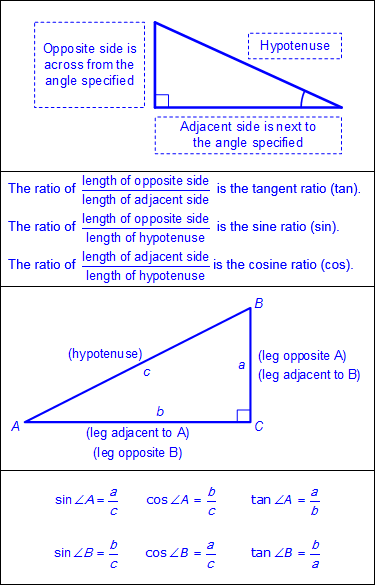• Right triangles
• Side lengths
• Angle measures
• Applications to real-world situations

Note(s):

• Middle School introduced ratios and unit rates when developing proportionality.
• Grade 8 uses the Pythagorean Theorem and its converse to solve problems.
• Geometry introduces trigonometric ratios.
• Various mathematical process standards will be applied to this student expectation as appropriate.
• TxCCRS
• II.A. Algebraic Reasoning – Identifying expressions and equations
• II.A.1. Explain the difference between expressions and equations.
• III.A. Geometric and Spatial Reasoning – Figures and their properties
• III.A.2. Form and validate conjectures about one-, two-, and three-dimensional figures and their properties.
• III.A.3. Recognize and apply right triangle relationships including basic trigonometry.
• III.B. Geometric and Spatial Reasoning – Transformations and symmetry
• III.B.1. Identify transformations and symmetries of figures.
• III.B.2. Use transformations to investigate congruence, similarity, and symmetries of figures.
• III.D. Geometric and Spatial Reasoning – Measurements involving geometry and algebra
• III.D.3. Determine indirect measurements of geometric figures using a variety of methods.
• VII.A. Problem Solving and Reasoning – Mathematical problem solving
• VII.A.1. Analyze given information.
• VII.A.3. Determine a solution.
• VII.B. Problem Solving and Reasoning – Proportional reasoning
• VII.B.1. Use proportional reasoning to solve problems that require fractions, ratios, percentages, decimals, and proportions in a variety of contexts using multiple representations.
• VIII.A. Communication and Representation – Language, terms, and symbols of mathematics
• VIII.A.3. Use mathematical language for reasoning, problem solving, making connections, and generalizing.
• IX.B. Connections – Connections of mathematics to nature, real-world situations, and everyday life
• IX.B.1. Use multiple representations to demonstrate links between mathematical and real-world situations.
G.9B Apply the relationships in special right triangles 30°-60°-90° and 45°-45°-90° and the Pythagorean theorem, including Pythagorean triples, to solve problems.

Apply

THE RELATIONSHIPS IN SPECIAL RIGHT TRIANGLES 30°–60°–90° AND 45°–45°–90° AND THE PYTHAGOREAN THEOREM, INCLUDING PYTHAGOREAN TRIPLES, TO SOLVE PROBLEMS

Including, but not limited to:

• 30°–60°–90° right triangle relationships – the hypotenuse is twice as long as the shorter leg, and the longer leg is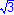times as long as the shorter leg.
• x represents the length of the short leg.
•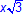represents the length of the longer leg.
• 2x represents the length of the hypotenuse.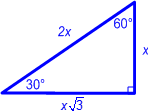• 45°–45°–90° right triangle relationships – the legs are equal in measure and the hypotenuse is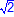times as long as a leg.
• x represents both congruent legs.
•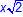represents the hypotenuse.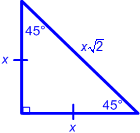• Pythagorean Theorem
• Pythagorean triples – three positive integers, a, b and c, such that a2 + b2 = c2, triples can be generated by multiplying a given Pythagorean triple by any positive integer
• Methods for finding Pythagorean triples
• Beginning with an odd integer
• Let a = an odd integer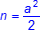b = integer to the left of n on the number line
c = integer to the right of n on the number line
• Beginning with an even integer
• Let a = an even integer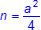b = integer to the left of n on the number line
c = integer to the right of n on the number line
• Solutions with rounded decimal answers
• Applications to real-world situations

Note(s):

• Grade 8 used the Pythagorean Theorem and its converse to solve problems.
• Geometry introduces special right triangles.
• Various mathematical process standards will be applied to this student expectation as appropriate.
• TxCCRS
• II.A. Algebraic Reasoning – Identifying expressions and equations
• II.A.1. Explain the difference between expressions and equations.
• III.A. Geometric and Spatial Reasoning – Figures and their properties
• III.A.2. Form and validate conjectures about one-, two-, and three-dimensional figures and their properties.
• III.A.3. Recognize and apply right triangle relationships including basic trigonometry.
• III.B. Geometric and Spatial Reasoning – Transformations and symmetry
• III.B.1. Identify transformations and symmetries of figures.
• III.B.2. Use transformations to investigate congruence, similarity, and symmetries of figures.
• III.D. Geometric and Spatial Reasoning – Measurements involving geometry and algebra
• III.D.3. Determine indirect measurements of geometric figures using a variety of methods.
• VII.A. Problem Solving and Reasoning – Mathematical problem solving
• VII.A.1. Analyze given information.
• VII.A.3. Determine a solution.
• VII.B. Problem Solving and Reasoning – Proportional reasoning
• VII.B.1. Use proportional reasoning to solve problems that require fractions, ratios, percentages, decimals, and proportions in a variety of contexts using multiple representations.
• VIII.A. Communication and Representation – Language, terms, and symbols of mathematics
• VIII.A.3. Use mathematical language for reasoning, problem solving, making connections, and generalizing.
• IX.B. Connections – Connections of mathematics to nature, real-world situations, and everyday life
• IX.B.1. Use multiple representations to demonstrate links between mathematical and real-world situations.
G.10 Two-dimensional and three-dimensional figures. The student uses the process skills to recognize characteristics and dimensional changes of two- and three-dimensional figures. The student is expected to:
G.10B

Determine and describe how changes in the linear dimensions of a shape affect its perimeter, area, surface area, or volume, including proportional and non-proportional dimensional change.

Determine, Describe

HOW CHANGES IN THE LINEAR DIMENSIONS OF A SHAPE AFFECT ITS PERIMETER, AREA, SURFACE AREA, OR VOLUME, INCLUDING PROPORTIONAL DIMENSIONAL CHANGE

Including, but not limited to:

• Verbal and written description
• Dimensional change
• Perimeter and circumference
• Area and surface area
• Volume
• Proportional change
• Three-dimensional proportional change – three dimensions multiplied by the same scale factor
• Comparison of the effect of proportional dimensional change
• Emphasis on connections to units
• Dimension changes in real-world problem situations

Note(s):

• Grade 7 and 8 modeled the effect on linear and area measurements of dilated two-dimensional shapes.
• Various mathematical process standards will be applied to this student expectation as appropriate.
• TxCCRS
• I.C. Numeric Reasoning – Systems of measurement
• I.C.1. Select or use the appropriate type of method, unit, and tool for the attribute being measured.
• III.A. Geometric and Spatial Reasoning – Figures and their properties
• III.A.2. Form and validate conjectures about one-, two-, and three-dimensional figures and their properties.
• III.B. Geometric and Spatial Reasoning – Transformations and symmetry
• III.B.1. Identify transformations and symmetries of figures.
• III.B.2. Use transformations to investigate congruence, similarity, and symmetries of figures.
• III.C. Geometric and Spatial Reasoning – Connections between geometry and other mathematical content strands
• III.C.1. Make connections between geometry and algebraic equations.
• III.D. Geometric and Spatial Reasoning – Measurements involving geometry and algebra
• III.D.1. Find the perimeter and area of two-dimensional figures.
• III.D.2. Determine the surface area and volume of three-dimensional figures.
• III.D.3. Determine indirect measurements of geometric figures using a variety of methods.
• VII.A. Problem Solving and Reasoning – Mathematical problem solving
• VII.A.1. Analyze given information.
• VIII.A. Communication and Representation – Language, terms, and symbols of mathematics
• VIII.A.3. Use mathematical language for reasoning, problem solving, making connections, and generalizing.
G.11 Two-dimensional and three-dimensional figures. The student uses the process skills in the application of formulas to determine measures of two- and three-dimensional figures. The student is expected to:
G.11B Determine the area of composite two-dimensional figures comprised of a combination of triangles, parallelograms, trapezoids, kites, regular polygons, or sectors of circles to solve problems using appropriate units of measure.

Determine

THE AREA OF COMPOSITE TWO-DIMENSIONAL FIGURES COMPRISED OF A COMBINATION OF TRIANGLES, PARALLELOGRAMS, TRAPEZOIDS, KITES, REGULAR POLYGONS, OR SECTORS OF CIRCLES TO SOLVE PROBLEMS USING APPROPRIATE UNITS OF MEASURE

Including, but not limited to:

• Composites of two-dimensional figures
• Triangles
• Parallelograms
• Trapezoids
• Kites
• Regular polygons
• Sectors of circles
• Applications to real-world situations
• Appropriate use of units of measure

Note(s):

• Previous grade levels used units, tools, and formulas to find the area of figures in problem situations.
• Previous grade levels introduced composites of two-dimensional figures.
• Geometry introduces kites, regular polygons, and sectors as shapes that can make up composite figures.
• Various mathematical process standards will be applied to this student expectation as appropriate.
• TxCCRS
• II.A. Algebraic Reasoning – Identifying expressions and equations
• II.A.1. Explain the difference between expressions and equations.
• III.D. Geometric and Spatial Reasoning – Measurements involving geometry and algebra
• III.D.1. Find the perimeter and area of two-dimensional figures.
• VII.A. Problem Solving and Reasoning – Mathematical problem solving
• VII.A.3. Determine a solution.
G.11C Apply the formulas for the total and lateral surface area of three-dimensional figures, including prisms, pyramids, cones, cylinders, spheres, and composite figures, to solve problems using appropriate units of measure.

Apply

THE FORMULAS FOR THE TOTAL AND LATERAL SURFACE AREA OF THREE-DIMENSIONAL FIGURES, INCLUDING PRISMS, PYRAMIDS, CONES, CYLINDERS, SPHERES, AND COMPOSITE FIGURES TO SOLVE PROBLEMS USING APPROPRIATE UNITS OF MEASURE

Including, but not limited to:

• Connections between formulas and models, including nets
• Formulas for lateral and total surface area
• Prisms
• Lateral surface area
• S = Perimeter of the base times the height
• S = Ph
• Total surface area
• S = Perimeter of the base times the height plus 2 times the area of the base
• S = Ph + 2B
• Pyramids
• Lateral surface area
• S =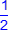times the perimeter of the base times the slant height
• S =P
• Total surface area
• S =times the perimeter of the base times the slant height plus the area of the base
• S =Pℓ + B
• Cones
• Lateral surface area
• S = π times the radius times the slant height
• S = πrℓ
• Total surface area
• S = π times the radius times the slant height plus the area of the base
• S = πrℓ +πr2
• Cylinders
• Lateral surface area
• S = 2 times π times the radius times the height
• S = 2πrh
• Total surface area
• S = 2 times π times the radius times the height plus 2 times the area of the base
• S = 2πrh + 2πr2
• Spheres
• Total surface area
• S = 4 times π times the radius squared
• S = 4πr2
• Composite figures
• Applications to real-world situations
• Use of appropriate units of measure

Note(s):

• Previous grade levels used units, tools, and formulas to find area of figures in problem situations.
• Previous grade levels introduced the terminology of regular polygons.
• Grade 7 determined the surface area of pyramids and prisms using nets.
• Grade 8 connects previous knowledge of surface area and nets to the surface area formulas.
• Geometry introduces cones, pyramids, spheres, and composite figures to the surface area formulas.
• Various mathematical process standards will be applied to this student expectation as appropriate.
• TxCCRS
• II.A. Algebraic Reasoning – Identifying expressions and equations
• II.A.1. Explain the difference between expressions and equations.
• III.D. Geometric and Spatial Reasoning – Measurements involving geometry and algebra
• III.D.2. Determine the surface area and volume of three-dimensional figures.
• VII.A. Problem Solving and Reasoning – Mathematical problem solving
• VII.A.3. Determine a solution.
G.11D Apply the formulas for the volume of three-dimensional figures, including prisms, pyramids, cones, cylinders, spheres, and composite figures, to solve problems using appropriate units of measure.

Apply

THE FORMULAS FOR THE VOLUME OF THREE-DIMENSIONAL FIGURES, INCLUDING PRISMS, PYRAMIDS, CONES, CYLINDERS, SPHERES, AND COMPOSITE FIGURES TO SOLVE PROBLEMS USING APPROPRIATE UNITS OF MEASURE

Including, but not limited to:

• Connections between formulas and models
• Formulas for volume
• Prisms
• V = Area of the base times the height
• V = Bh
• Pyramids
• V =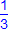times the area of the base times the height
• V =Bh
• Cylinders
• V = Area of the base times the height
• V = πr2h
• Cones
• V =times the area of the base times the height
• V =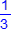Bh
• Spheres
• V =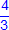times π times the radius cubed
• V =πr3
• Composite figures
• Applications to real-world situations
• Use of appropriate units of measure

Note(s):

• Grades 7 and 8 introduced determining and applying formulas to solve problems involving lateral and total surface area and volume of three-dimensional figures.
• Various mathematical process standards will be applied to this student expectation as appropriate.
• TxCCRS
• II.A. Algebraic Reasoning – Identifying expressions and equations
• II.A.1. Explain the difference between expressions and equations.
• III.D. Geometric and Spatial Reasoning – Measurements involving geometry and algebra
• III.D.2. Determine the surface area and volume of three-dimensional figures.
• VII.A. Problem Solving and Reasoning – Mathematical problem solving
• VII.A.3. Determine a solution.
G.12 Circles. The student uses the process skills to understand geometric relationships and apply theorems and equations about circles. The student is expected to:
G.12B Apply the proportional relationship between the measure of an arc length of a circle and the circumference of the circle to solve problems.

Apply

THE PROPORTIONAL RELATIONSHIP BETWEEN THE MEASURE OF AN ARC LENGTH OF A CIRCLE AND THE CIRCUMFERENCE OF THE CIRCLE TO SOLVE PROBLEMS

Including, but not limited to:

• Arc length of a circle – a fractional distance of the circumference of a circle defined by the arc
• Measure of arc length of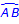is denoted as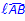• Connecting the proportional relationshipor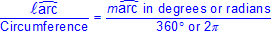• Applications to real-world problem situations
• Use of appropriate units of measure
• Use of various tools
• Protractor and straightedge
• Dynamic geometric software
• Patty paper

Note(s):

• Previous grade levels explored characteristics of circles and proportional relationships.
• Various mathematical process standards will be applied to this student expectation as appropriate.
• TxCCRS
• II.A. Algebraic Reasoning – Identifying expressions and equations
• II.A.1. Explain the difference between expressions and equations.
•  III.A. Geometric and Spatial Reasoning – Figures and their properties
• III.A.2. Form and validate conjectures about one-, two-, and three-dimensional figures and their properties.
• III.C. Geometric and Spatial Reasoning – Connections between geometry and other mathematical content strands
• III.C.1. Make connections between geometry and algebraic equations.
• III.D. Geometric and Spatial Reasoning – Measurements involving geometry and algebra
• III.D.1. Find the perimeter and area of two-dimensional figures.
• III.D.3. Determine indirect measurements of geometric figures using a variety of methods.
• VII.A. Problem Solving and Reasoning – Mathematical problem solving
• VII.A.1. Analyze given information.
• VII.A.3. Determine a solution.
• VII.B. Problem Solving and Reasoning – Proportional reasoning
• VII.B.1. Use proportional reasoning to solve problems that require fractions, ratios, percentages, decimals, and proportions in a variety of contexts using multiple representations.
• IX.B. Connections – Connections of mathematics to nature, real-world situations, and everyday life
• IX.B.1. Use multiple representations to demonstrate links between mathematical and real-world situations.
G.12C Apply the proportional relationship between the measure of the area of a sector of a circle and the area of the circle to solve problems.

Apply

THE PROPORTIONAL RELATIONSHIP BETWEEN THE MEASURE OF THE AREA OF A SECTOR OF A CIRCLE AND THE AREA OF THE CIRCLE TO SOLVE PROBLEMS

Including, but not limited to:

• Sector of a circle – a region of the circle bounded by a central angle and its intercepted arc
• Connecting the proportional relationship• Applications to real-world problem situations
• Use of appropriate units of measure
• Use of various tools
• Protractor and straightedge
• Dynamic geometric software
• Patty paper

Note(s):

• Previous grade levels explored characteristics of circles and proportional relationships.
• Various mathematical process standards will be applied to this student expectation as appropriate.
• TxCCRS
• II.A. Algebraic Reasoning – Identifying expressions and equations
• II.A.1. Explain the difference between expressions and equations.
• III.A. Geometric and Spatial Reasoning – Figures and their properties
• III.A.2. Form and validate conjectures about one-, two-, and three-dimensional figures and their properties.
• III.C. Geometric and Spatial Reasoning – Connections between geometry and other mathematical content strands
• III.C.1. Make connections between geometry and algebraic equations.
• III.D. Geometric and Spatial Reasoning – Measurements involving geometry and algebra
• III.D.1. Find the perimeter and area of two-dimensional figures.
• III.D.3. Determine indirect measurements of geometric figures using a variety of methods.
• VII.A. Problem Solving and Reasoning – Mathematical problem solving
• VII.A.1. Analyze given information.
• VII.A.3. Determine a solution.
• VII.B. Problem Solving and Reasoning – Proportional reasoning
• VII.B.1. Use proportional reasoning to solve problems that require fractions, ratios, percentages, decimals, and proportions in a variety of contexts using multiple representations.
• IX.B. Connections – Connections of mathematics to nature, real-world situations, and everyday life
• IX.B.1. Use multiple representations to demonstrate links between mathematical and real-world situations.